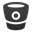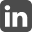Armand Halbert
Customer Success Engineer
DataKitchen

Contact:
armand.halbert@gmail.com
ajhalber@us.ibm.com
Resume - pdf
Resume - textPublished on:
Tuesday, October 29, 2013

# On Sorting Badly

Recently, I saw this hilarious StackOverflow post: How bad a sorting algorithm can you make? Apart from the jokes, me and a few friends set out to make the worse algorithm we could in these constraints.

• Must have a finite upper bound - i.e. not $O(\infty)$
• Cannot be random - otherwise it’s bound is $O(\infty)$
• Must always sort the array correctly

Since MonkeySort(generate all permutations of an array) runs in $O(n*n!)$ we thought generating all possible arrays of size n would be worse. Imagine my surprise to find this was not the case.

We can verify if an array is sorted and has the right data in $O(n)$, and there are $(2^m)^n$ possible arrays, where m is the bit size of a word on your computer. So, in the worse case, this will run in $O(n(2^m)^n)$

So…which runs faster?

$\lim \limits_{n \to \infty} \frac{n(2^m)^n}{n!} = 0$ for any value of m you care to have.

How could this be? Surely the set of all permutations of an array A of size $n$ is a subset of the set of all possible arrays of size $n$? This statement is true - however we are forgetting that the $O(n!)$ will create duplicate arrays, especially at large $n$. So generating all possible arrays of size $n$ is still better because we do not make duplicates.

Even generating all arrays of any possible size is still better than generating all permutations.

$\lim \limits_{n \to \infty} \frac{ \sum_{a=1}^n a(2^m)^a}{n!} = 0$ for any m. While this is an interesting result, it’s the first time I’ve ever been disturbed by a math answer. I’m still working on an algorithm that runs in $O(n^n)$ time.

<< Previous